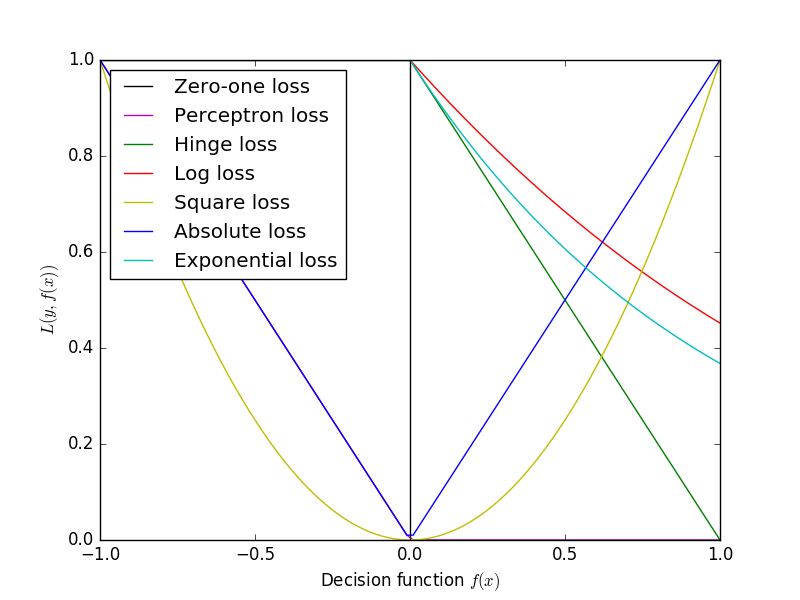机器学习中常见的损失函数

机器学习中常见的损失函数

一般来说，我们在进行机器学习任务时，使用的每一个算法都有一个目标函数，算法便是对这个目标函数进行优化，特别是在分类或者回归任务中，便是使用损失函数（Loss Function）作为其目标函数，又称为代价函数(Cost Function)。
损失函数是用来评价模型的预测值$\stackrel{^}{Y}=f\left(X\right)$$\hat{Y}=f(X)$与真实值$Y$$Y$的不一致程度，它是一个非负实值函数。通常使用$L\left(Y,f\left(x\right)\right)$$L(Y, f(x))$来表示，损失函数越小，模型的性能就越好。
设总有$N$$N$个样本的样本集为$\left(X,Y\right)=\left({x}_{i},{y}_{i}\right)$$(X,Y)={(x_i,y_i)}$${y}_{i},i\in \left[1,N\right]$$y_i, i \in [1,N]$为样本$i$$i$的真实值，$\stackrel{^}{{y}_{i}}=f\left({x}_{i}\right),i\in \left[1,N\right]$$\hat{y_i}=f(x_i), i \in [1,N]$为样本$i$$i$的预测值，$f$$f$为分类或者回归函数。

$L=\sum _{i=1}^{N}\ell \left({y}_{i},\stackrel{^}{{y}_{i}}\right)$

常见的损失函数$\ell \left({y}_{i},\stackrel{^}{{y}_{i}}\right)$$\ell(y_i,\hat{y_i})$有以下几种：

Zero-one Loss

Zero-one Loss即0-1损失，它是一种较为简单的损失函数，如果预测值与目标值不相等，那么为1，否则为0，即：

$\begin{array}{}\text{(1)}& \ell \left({y}_{i},\stackrel{^}{{y}_{i}}\right)=\left\{\begin{array}{ll}1,& {y}_{i}\ne \stackrel{^}{{y}_{i}}\\ 0,& {y}_{i}=\stackrel{^}{{y}_{i}}\end{array}\end{array}$

Perceptron Loss

Perceptron Loss即为感知损失。即：

$\begin{array}{}\text{(2)}& \ell \left({y}_{i},\stackrel{^}{{y}_{i}}\right)=\left\{\begin{array}{ll}1,& |{y}_{i}-\stackrel{^}{{y}_{i}}|>t\\ 0,& |{y}_{i}-\stackrel{^}{{y}_{i}}|\le t\end{array}\end{array}$

Hinge Loss

Hinge损失可以用来解决间隔最大化问题，如在SVM中解决几何间隔最大化问题，其定义如下：

$\ell \left({y}_{i},\stackrel{^}{{y}_{i}}\right)=max\left\{0,1-{y}_{i}\cdot \stackrel{^}{{y}_{i}}\right\}$

${y}_{i}\in \left\{-1,+1\right\}$

Log Loss

$\ell \left({y}_{i},\stackrel{^}{{y}_{i}}\right)=-{y}_{i}\cdot log\stackrel{^}{{y}_{i}}-\left(1-{y}_{i}\right)\cdot log\left(1-\stackrel{^}{{y}_{i}}\right)$

${y}_{i}\in \left\{0,1\right\}$

$0\cdot log\cdot =0$

Square Loss

Square Loss即平方误差，常用于回归中。即：

$\ell \left({y}_{i},\stackrel{^}{{y}_{i}}\right)=\left({y}_{i}-\stackrel{^}{{y}_{i}}{\right)}^{2}$

${y}_{i},\stackrel{^}{{y}_{i}}\in \mathrm{\Re }$

Absolute Loss

Absolute Loss即绝对值误差，常用于回归中。即：

$\ell \left({y}_{i},\stackrel{^}{{y}_{i}}\right)=|{y}_{i}-\stackrel{^}{{y}_{i}}|$

${y}_{i},\stackrel{^}{{y}_{i}}\in \mathrm{\Re }$

Exponential Loss

$\ell \left({y}_{i},\stackrel{^}{{y}_{i}}\right)=exp\left(-{y}_{i}\cdot \stackrel{^}{{y}_{i}}\right)$

${y}_{i}\in \left\{-1,1\right\}$

正则

$L=\sum _{i=1}^{N}\ell \left({y}_{i},\stackrel{^}{{y}_{i}}\right)+\lambda \cdot R\left(\omega \right)$

各损失函数图形如下：